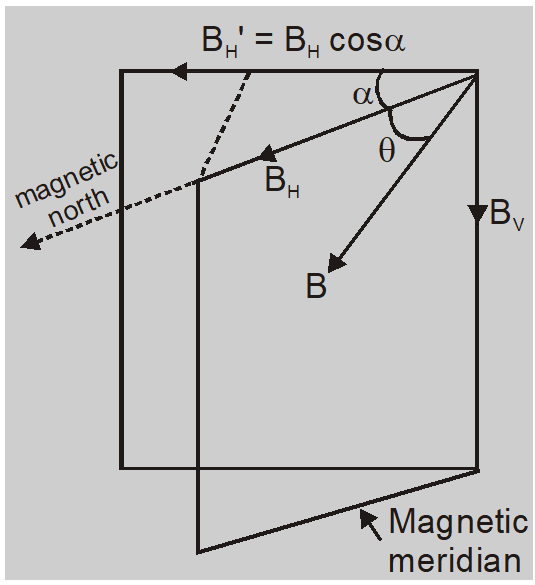Most Affordable JEE | NEET | 8,9,10 Preparation by Kota's Top IITian Doctor Faculties

# True Dip and Apparent Dip - Magnetism and Matter Class 12Here we will study about True Dip and Apparent Dip.

## Apparent Dip

The dip at a place is determined by a dip circle. It consists of a magnetized needle capable of rotation in a vertical plane about a horizontal axis. The needle moves over a vertical scale graduated in degrees.

If the plane of the scale of the dip circle is not in the magnetic meridian then the needle will not indicate the correct direction of the earth's magnetic field. The angle made by the needle with the horizontal is called Apparent Dip.

## True Dip

When the plane of scale of the dip circle is in the magnetic meridian the needle comes to rest in direction of the earth's magnetic field. The angle made by the needle with the horizontal is called True Dip.

Suppose dip circle is set at angle $\alpha$ to the magnetic meridian.

Horizontal component $${B_H}' = {B_H}\cos \alpha$$

Vertical component ${B_V}' = {B_V}$ (remains unchanged)

Apparent dip is ${\theta ^\prime }\tan {\theta ^\prime } = {{{B_V}'} \over {{B_H}'}} = {{{B_V}} \over {{B_H}\cos \alpha }} = {{\tan \theta } \over {\cos \alpha }}\left( {\tan \theta = {{{B_V}} \over {{B_H}}} = {\rm{ true dip }}} \right)$1. For a vertical plane other than magnetic meridian $\alpha>0$ or $\cos \alpha<1$ so $\theta^{\prime}>\theta$ In a vertical plane other than magnetic meridian angle of dip is more than in magnetic meridian.
2. For a plane perpendicular to magnetic meridian $\alpha=\frac{\pi}{2}$ $\therefore \tan \theta^{\prime}=\infty \quad$ so $\quad \theta^{\prime}=\frac{\pi}{2}$ So in a plane perpendicular to magnetic meridian dip needle will become vertical.

### At magnetic equator :

1. The angle of dip is zero.
2. Vertical component of earths magnetic field becomes zero $B_{V}=B \sin \theta=B \sin 0=0$
3. A freely suspended magnet will become horizontal at the magnetic equator.
4. At the equator earth's magnetic field is parallel to the earth's surface i.e., horizontal.

### At magnetic poles :

1. The angle of dip is $90^{\circ}$
2. Horizontal component of earth's magnetic field becomes zero. $B_{H}=B \cos \theta=B \cos 90=0$
3. A freely suspended magnet will become vertical at magnetic poles.
4. At poles, the earth's magnetic field is perpendicular to the surface of the earth i.e. vertical.

Ex. If $\theta_{1}$ and $\theta_{2}$ are angles of dip in two vertical planes at right angle to each other and $\theta$ is true dip then prove $\cot ^{2} \theta=\cot ^{2} \theta_{1}+\cot ^{2} \theta_{2}$.

Sol. If the vertical plane in which dip is $\theta_{1}$ subtends an angle $\alpha$ with meridian than other vertical plane in which dip is $\theta_{2}$ and is perpendicular to first will make an angle of $90-\alpha$ with magnetic meridian. If $\theta_{1}$ and $\theta_{2}$ are apparent dips than

$\tan \theta_{1}=\frac{B_{V}}{B_{H} \cos \alpha}$

$\tan \theta_{2}=\frac{B_{V}}{B_{H} \cos (90-\alpha)}=\frac{B_{V}}{B_{H} \sin \alpha}$

$\cot ^{2} \theta_{1}+\cot ^{2} \theta_{2}=\frac{1}{\left(\tan \theta_{1}\right)^{2}}+\frac{1}{\left(\tan \theta_{2}\right)^{2}}$

$=\frac{B_{H}^{2} \cos ^{2} \alpha+B_{H}^{2} \sin ^{2} \alpha}{B_{V}^{2}}=\frac{B_{H}^{2}}{B_{V}^{2}}$

$=\left(\frac{B \cos \theta}{B \sin \theta}\right)^{2}=\cot ^{2} \theta$

So $\quad \cot ^{2} \theta_{1}+\cot ^{2} \theta_{2}=\cot ^{2} \theta$

At eSaral we are offering a complete platform for IIT-JEE & NEET preparation. The main mission behind eSaral is to provide education to each and every student in India by eliminating the Geographic and Economic factors, as a nation’s progress and development depends on the availability of quality education to each and every one. With the blend of education & technology, eSaral team made the learning

pharmacy uk
Oct. 25, 2023, 6:35 a.m.
Hi, i think that i saw you visited my website thus i came to “return the favor”.I am attempting to find things to improve my web site!I suppose its ok to use some of your ideas!!
Ashmit Negi
March 19, 2023, 6:35 a.m.
This was very helpful. I could not find a simple formula for this anywhere except here and now I can smoothly cruize through the questions i couldn't solve earlier.
Praveen saini
Sept. 2, 2021, 2:09 p.m.
Good
Srijan Srivastava
Aug. 16, 2021, 7 p.m.
Really, help full!!
( ͡° ͜ʖ ͡°)
July 23, 2021, 12:36 a.m.
Came in JEE mains 2021July
Patidar
June 16, 2021, 11:09 a.m.